One of the possible reasons the execution of a DAX expression can be slower, is the presence of nested iterators. The real issue is not the presence of an iterator in and of itself, but the cardinality of the materialization required by the lowest level of context transition. While it is true that moving most of the workload to the storage engine is a good idea, finding the right balance between materialization and storage engine calculation is the ultimate goal.

For example, consider the following data model of a sample Contoso database, which contains one million rows in the Sales table.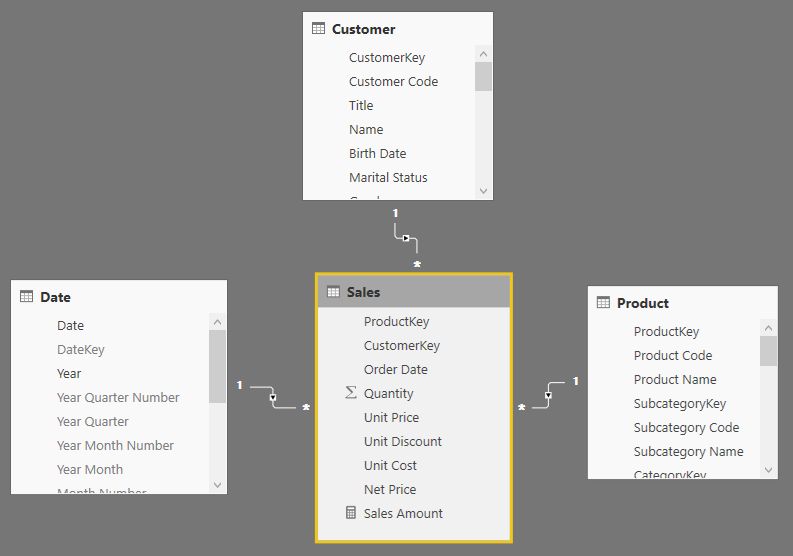The Gross Amount measure is defined as follows:

```Gross Amount :=
SUMX (
Sales,
Sales[Quantity] * Sales[Unit Price]
)
```

Both Product and Customer have a discount that should be applied to the Gross Amount measure. Thus, an intuitive way to solve the problem is the following:

```Sales Amount Slow :=
SUMX (
Customer,
SUMX (
'Product',
VAR DiscountedProduct = 1 - 'Product'[Product Discount]
VAR DiscountedCustomer = 1 - Customer[Customer Discount]
RETURN
[Gross Amount]
* DiscountedProduct
* DiscountedCustomer
)
)
```

The Sales Amount Slow measure produces the following result, which is correct.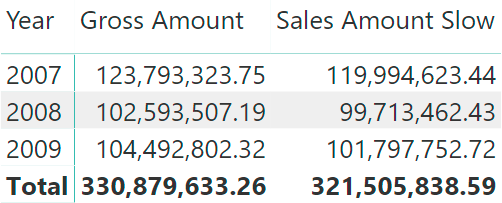The Sales Amount Slow measure is easy to read, but it is also the slowest version. Analyzing the query produced by the previous report with DAX Studio, we isolated the Sales Amount Slow measure. This calculation generates large materializations up to 239,684 rows, which is the number of existing combinations of customers and products in the Sales table.

```EVALUATE
SUMMARIZECOLUMNS (
"Sales_Amount", [Sales Amount Slow]
)
ORDER BY
[IsGrandTotalRowTotal] DESC,
'Date'[Year]
```

Materialization occurs because of the context transition of the Gross Amount measure referenced within Sales Amount Slow. In fact, expanding Gross Amount in the Sales Amount Slow measure would produce this equivalent measure:

```Sales Amount Slow Expanded :=
SUMX (
Customer,
SUMX (
'Product',
VAR DiscountedProduct = 1 - 'Product'[Product Discount]
VAR DiscountedCustomer = 1 - Customer[Customer Discount]
RETURN
CALCULATE (
SUMX (
Sales,
Sales[Quantity] * Sales[Unit Price]
)
) * DiscountedProduct * DiscountedCustomer
)
)
```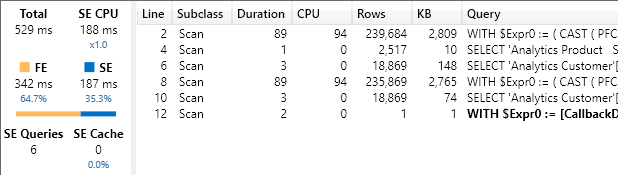The expanded code of Sales Amount Slow Expanded clarifies that there are actually three nested iterators and not just two. Only the innermost iterator pushes the evaluation into the storage engine, whereas the other iterators must be executed by the formula engine. In other words, the formula engine iterates the result of the Gross Amount measure computed by the storage engine – not because it is a measure, but because it is the innermost aggregation that can be performed by the storage engine.

An alternative approach would be that of removing any context transition in the calculation, creating a single iterator and relying on the RELATED function to retrieve the discount of product and customer for each transaction in Sales.

```Sales Amount Intermediate :=
SUMX (
Sales,
VAR LineAmount = Sales[Quantity] * Sales[Unit Price]
VAR ProductDiscount =
RELATED ( 'Product'[Product Discount] )
VAR CustomerDiscount =
RELATED ( 'Customer'[Customer Discount] )
RETURN
LineAmount
* ( 1 - ProductDiscount )
* ( 1 - CustomerDiscount )
)
```

The Sales Amount Intermediate measure produces the same result as Sales Amount Slow as long as the underlying data is a floating point value (Decimal Number in Power BI). In cases where the data model uses a currency data type (Fixed Decimal Number in Power BI) there can be differences in the result. Indeed, values may be rounded differently at various steps of the calculation.

The performance of the Sales Amount Intermediate measure is better, as shown in the following DAX Studio screenshot.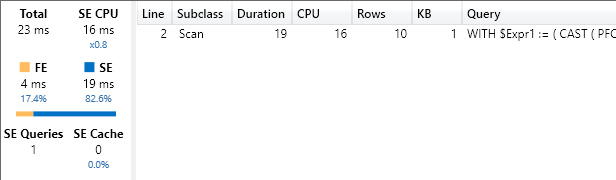However, this approach poses two issues. The biggest is that the business logic defined in the Gross Amount measure must be duplicated within the Sales Amount Intermediate measure – that is, the code duplicated in the LineAmount variable. The second issue is that the presence of different data types in the data model, or the presence of more complex calculations might generate a CallbackDataID for each row of the Sales table.

For example, the following is the result observed in DAX Studio in case the Unit Price data type is a currency instead of a floating point. This condition can be obtained in Power BI by changing the data type from Decimal Number to Fixed Decimal Number, but keep in mind that in this case the result of the calculation will be different because of rounding differences.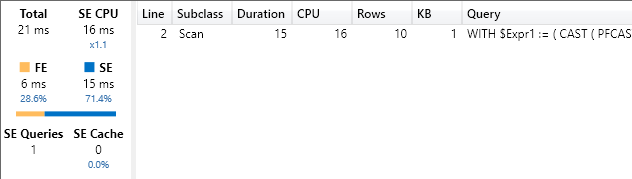The optimal approach is a balanced one. The materialization caused by the slowest measure depends on the cardinality of the iterators. Whenever possible, the cardinality should correspond to the one required by the terms used in the calculation, instead of simply iterating the tables where those terms are stored. In our calculation, we only need one column from each of the Customer and Product tables. Therefore, it is possible to rewrite the code by materializing the data for all the transactions with the same discount percentage, instead of materializing by products and customers that might have the same discount.

```Sales Amount Optimal :=
SUMX (
VALUES ( Customer[Customer Discount] ),
SUMX (
VALUES ( 'Product'[Product Discount] ),
VAR DiscountedProduct = 1 - 'Product'[Product Discount]
VAR DiscountedCustomer = 1 - Customer[Customer Discount]
RETURN
[Gross Amount]
* DiscountedProduct
* DiscountedCustomer
)
)
```

The Sales Amount Optimal measure might not have the same efficiency as Sales Amount Intermediate when used with the floating point number data type in Unit Price (data type set to Decimal Number in Power BI). This is because it generates two storage engine queries instead of one; however, it is usually very close in efficiency and does not require duplicating the business logic of the Gross Amount measure.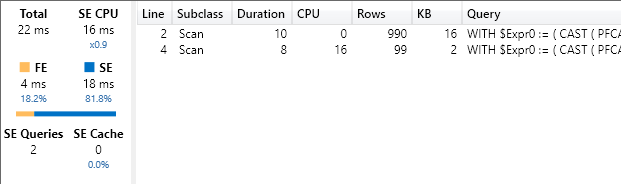Yet, in case there is a currency data type in Unit Price (data type set to Fixed Decimal Number in Power BI) the performance of the Sales Amount Optimal measure does not change. It remains faster than the Sales Amount Intermediate measure for the same data type. Moreover, with a currency data type the Sales Amount Optimal measure also produces a number much closer if not identical to the one of Sales Amount Slow, because of the reduction in rounding differences.

## Conclusions

Nested iterators in DAX might present performance issues if the combined cardinality of the iterators is large. Only the innermost iterator can be pushed to the storage engine, and oftentimes this iterator is “hidden” in a measure. The best practice is to reduce the cardinality of iterators that invoke measures so that they always minimize the materialization required to the storage engine.